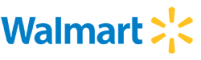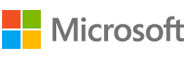New update is available. Click here to update.
Topics

# Find Pattern in String - KMP Algorithm

Easy0/40
Average time to solve is 10m+5 more companies

## Problem statement

You are given two strings 'S' and 'P' consisting of lowercase English alphabets. Your task is to find whether the 'P' is present in 'S' as a substring or not.

Note
``````1. There may be more than one occurrence of 'P' in 'S'.
2. Some alphabets in the strings may be repeated.
``````
Detailed explanation ( Input/output format, Notes, Images )
Constraints:
``````1 <= T <= 100
1 <= |S| <= 10000
1 <= |P| < |S|

Where |S| and |P| represents the length of the string 'S' and 'P' respectively.

Time limit: 1 sec.
``````

#### Sample input 1:

``````3
xxy yxxyxxy
a baac
cfg cgfgfc
``````

#### Sample output 1

``````YES
YES
NO
``````

#### Explanation for sample output 1

``````In the first test case, there are two substrings equal to P on index 1 and 4 in S.

In the second test case, there are two substrings equal to P on indexes 1 and 2 in S.
In the third test case, P does not exist in S.
``````

#### Sample input 2:

``````3
bbb abba
iqw hdhhdqoa
car caribbean
``````

#### Sample output 2

``````NO
NO
YES
``````

#### Explanation for sample output 2

`````` In the first test case, P does not exist in S.
In the second test case, P does not exist in S.
In the third test case, there is one substring equal to P on index 1 in S.
``````Console